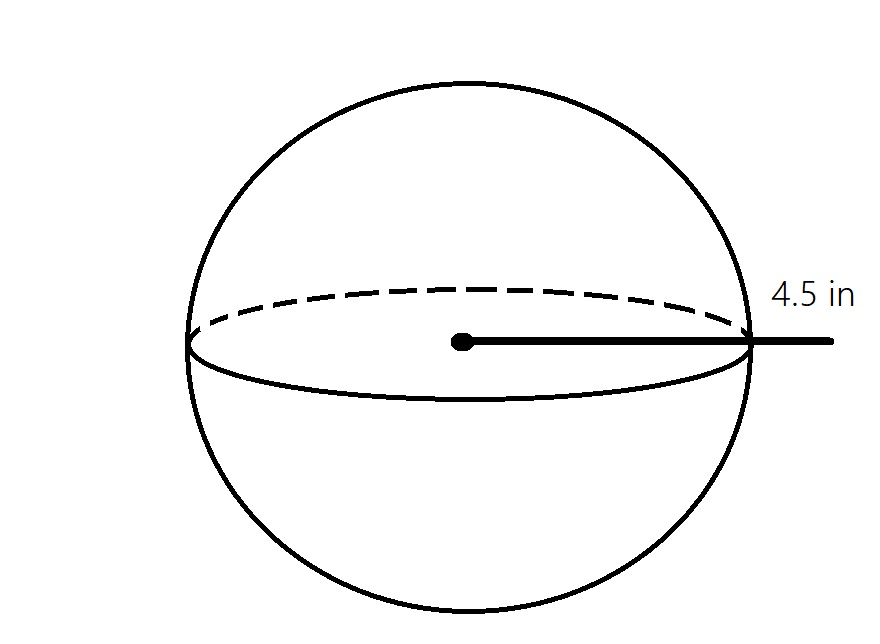kloseyq

2021-12-19

Determine how much air could fit inside a soccer ball with radius of 4.5 inches. Use 3.14 for $\pi$Alex Sheppard

Step 1
That mean we have to find the volume of the Soccer ball.
Since it is a Shere with radius

$\therefore$ volume of Soccer ball $=\frac{4}{3}⊼{r}^{3}$
$V=\frac{4}{3}×\stackrel{―}{\wedge }×{\left(4.5\right)}^{3}$
$=\frac{4}{3}×\stackrel{―}{\wedge }×91.128$
$=\frac{4}{3}×3.14×91.725$
$=\frac{4×286.1325}{3}$
$=\frac{1144.53}{3}$poleglit3

Step 1
The formula for a sphere is
$V=\frac{4}{3}\left(3.14\right\}\left({4.5}^{3}\right)$
When you calculate that, you get.
$\frac{243\left(3.14\right)}{2}$
Now you divide by 2.
$=381.7$

Do you have a similar question?